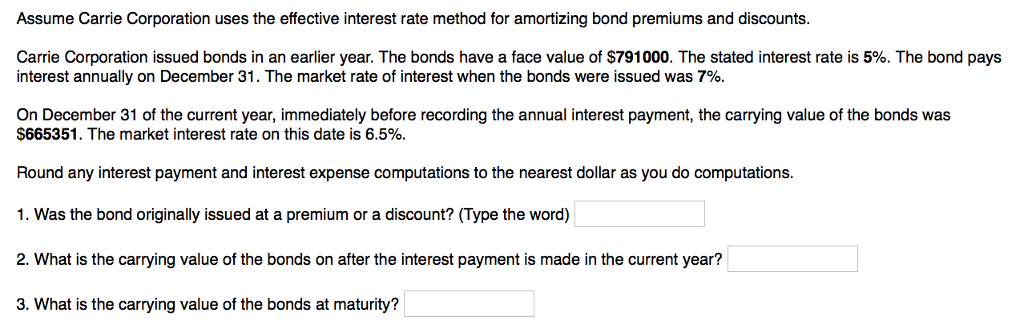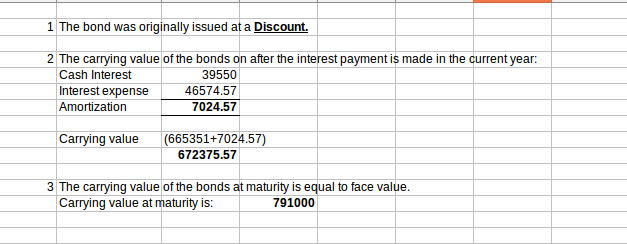# Question & Answer: Assume Carrie Corporation uses the effective interest rate method for amortizing bond premiums and discounts. Carrie…..Assume Carrie Corporation uses the effective interest rate method for amortizing bond premiums and discounts. Carrie Corporation issued bonds in an earlier year. The bonds have a face value of \$791000. The stated interest rate is 5%. The bond pays interest annually on December 31. The market rate of interest when the bonds were issued was 7%. On December 31 of the current year, immediately before recording the annual interest payment, the carrying value of the bonds was \$665351. The market interest rate on this date is 6.5%. Round any interest payment and interest expense computations to the nearest dollar as you do computations. 1. Was the bond originally issued at a premium or a discount? (Type the word) 2. What is the carrying value of the bonds on after the interest payment is made in the current year? 3. What is the carrying value of the bonds at maturity?